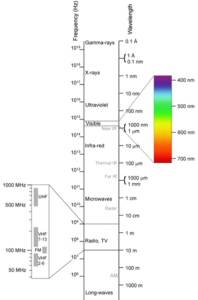## Putting It Together: Algebra EssentialsThe electromagnetic spectrum with the visible light range enlarged.

At the beginning of this module, you read that mathematics is the language of science. You also discovered that additional numbers were developed to deal with real-world applications, including the existence of nothing and debt. Fortunately, the sets and the formats of numbers has continued to develop so that scientists and other researchers have the mathematical tools they need to investigate the natural world.

It just so happens that you need a specific number format to describe the light you are using to read these words—scientific notation. Light is a form of energy transferred as an electromagnetic (EM) wave. X-rays, microwaves, and radio waves are other forms of EM waves. They are differentiated by their properties of wavelength, which is the distance from one part of a wave to the same part of the next wave, and frequency, which is the number of waves that pass a point in a given amount of time. The arrangement of EM waves in order of wavelength and frequency is known as the electromagnetic spectrum. You can see from this example, that visible light makes up only a very small portion of the spectrum.

So what is the difference between blue light and red light? If you look at the diagram, you see that blue light is labeled at about 420 nanometers (nm). Writing this out as meters gives  $4.20\times10^{-7}$m —that’s really small. Red light, meanwhile, is about 700nm or $\left(7.0\times10^{-7}\right)$m. That small difference has a huge impact on what you see.

The interesting thing about EM waves is that they all travel at the same speed in the vacuum of space—  $3.0\times10^8$m/s. So you can calculate either wavelength or frequency if you know the other.

$v=f\lambda$

where,

$v$ = speed (velocity)

$f$ = frequency

$\lambda$ = wavelength

For example, let’s find the frequency of red light with a wavelength of $7.0\times10^{-7}$m. First, rearrange to formula to solve for frequency. Then substitute in the given information.

\begin{align}f&=\frac{v}{\lambda} \\[1mm] &=\frac{3.0\times10^8m/s} {7.0\times10^{-7}m}\\[1mm] &=4.0\times10^{14}s^{-1}\end{align} Hertz

You can see that wavelength and frequency are inversely related. So blue light, which has a smaller wavelength than red light, will have a greater frequency if traveling at the same speed.

So what does it matter if you can calculate the frequency of light? It just so happens that you may be able to predict the future of the universe! If a wave is produced by one object and observed by another, the frequency of the wave changes if either the source of the wave or the observer move relative to the other. This is true for sound waves as well, and you may already be familiar with this phenomenon if you have listened to a siren or train whistle as it approaches and  passes you. When it comes to sound, frequency determines pitch so the sound gets higher as it approaches and lower as it moves away. This is known as the Doppler effect.

Now back to the universe. Objects in the universe, such as stars, give off light. Suppose you are an astronomer on Earth. If the source of light is moving toward Earth, the frequency of the light will increase. An increase in frequency means that it shifts toward the blue end of the spectrum, and is appropriately known as a blueshift. If the source of light is moving away from Earth, the frequency will decrease and shifts toward the red end of the spectrum. This shift, as you may have guessed, is a redshift.

Astronomers have used their understanding of the Doppler effect to make measurements regarding light from distant galaxies. As a result, they have learned that galaxies are moving apart from one another and the universe is expanding. Furthermore, the speed at which an object moves away is proportional to its distance. Because of the tremendous distances in space and the fast speed of light, none of this would be possible without being able to use formulas involving scientific notation.